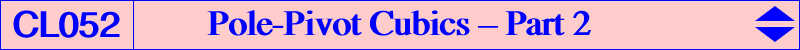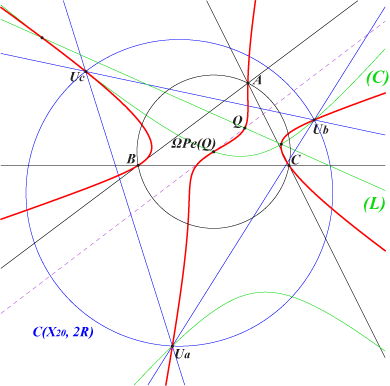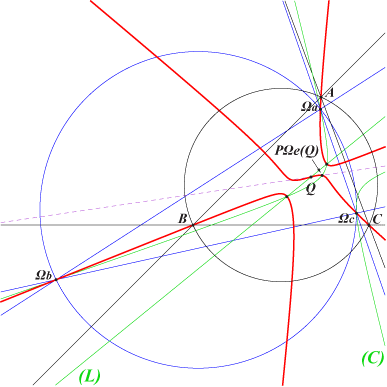Let us consider a pivotal cubic pK(Ω,P) with pole Ω = (p:q:r) and pivot P = (u:v:w). pK is an equilateral cubic (a pK60) if and only if – either its pivot P is the transform of its pole Ω under the mapping ΩPe : Ω -> P, – or its pole Ω is the transform of its pivot P under the mapping PΩe : P -> Ω. See Special Isocubics § 6.4 for more informations and constructions. These two mappings have the same fixed points namely A, B, C and X(11058), the isotomic conjugate of X(11057) = X(2)X(187) /\ X(30)X(76). Each mapping has three singular points : – for PΩe : Ua, Ub, Uc lying on a large number of curves. See Special Isocubics § 6.5, Table 18 and K001. – for ΩPe : Ωa, Ωb, Ωc lying on the PΩe–images of the curves above – K095, K307, K485 for instance – and also the PΩe–image of any line. These six points are not ruler and compass constructable but one can find several conics containing them. See Table 18 for Ua, Ub, Uc and Table 41 for Ωa, Ωb, Ωc. It follows that there are infinitely many pK60s with pivot Ua and pole on the line ΩbΩc, also infinitely many pK60s with pole Ωa and pivot on the line UbUc, and similarly for the other points. *** ΩPe transforms a line (L) into a conic (C) passing through Ua, Ub, Uc and PΩe transforms (L) into a conic (C') passing through Ωa, Ωb, Ωc. When (L) passes through a fixed point Q, the locus of intersections of (L) and (C) is a cubic Ke(Q) and the locus of intersections of (L) and (C') is a cubic K'e(Q). The cubics Ke(Q)Ke(Q) is a circumcubic passing through the points Ua, Ub, Uc, X(11058) as above, Q and ΩPe(Q). Ke(Q) is : – a K0 when Q lies on the Euler line, – circular if and only if Q = X(187) giving K330, – equilateral if and only if Q = G giving K142 See also K488.The cubics K'e(Q)K'e(Q) is a circumcubic passing through the points Ωa, Ωb, Ωc, X(11058) as above, Q and PΩe(Q). Note that Ke(Q) and K'e(Q) generate a pencil of cubics that contains the pivotal cubic with pivot X(11058) and isopivot Q. K'e(Q) is : – a K0 when Q lies on the Euler line, – circular for one point Q with rather complicated coordinates, – equilateral if and only if Q = G giving K485